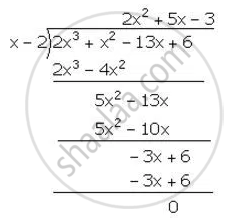Share

# Using the Remainder Theorem, Factorise Each of the Following Completely. 2x3 + X2 – 13x + 6 - Mathematics

Course

#### Question

Using the Remainder Theorem, factorise each of the following completely.

2x3 + x2 – 13x + 6

#### Solution

Let f(x) = 2x^3+x^2-13x+6

for x=2

Factors of constant term 6 are ≠1, ≠ 2, ≠ 3, ≠ 6.
Putting x = 2, we have:

f(x)=f(2) =2(2)^3+(2)^2-13(2)+6=16+4-26+6=0

Hence, (x-2) is a factor of f (x)∴ 2x^3+x^2-13x+6=(x-2)(2x^2+5x-3)

= (x-2)(2x^2+6x-x-3)

= (x-2)[2x(x-3)-(x+3)]

=(x-2)(x+3)(2x-1)

Is there an error in this question or solution?